# gateR: Flow/Mass Cytometry Gating via Spatial Kernel Density Estimation

#### 2020-11-20

The gateR package is a suite of R functions to identify significant spatial clustering of mass and flow cytometry data used in immunological investigations. The gateR package can be used for a panel of all surface markers, or a mixture of surface markers and functional read outs. The gateR package performs a gating technique that estimates statistically significant marker combination values within which one immunologically distinctive group (i.e., disease case) is more associated than another group (i.e., healthy control), successively, using various combinations (i.e., “gates”) of markers to examine features of cells that may be different between groups. For a two-group comparison, the gateR package uses the spatial relative risk function that is estimated using the sparr package. The gates are conducted in two-dimensional space comprised of two markers.

Examples of a single condition with two groups:

1. Disease case v. healthy control
2. Time 2 v. Time 1 (baseline)

For a two-group comparison of two conditions we estimate two relative risk surfaces for one condition and then a ratio of the relative risks. For example:

1. Estimate a relative risk surface for:
1. Condition 2B v. Condition 2A
2. Condition 1B v. Condition 1A
2. Estimate relative risk surface for the ratio:

$\frac{(\frac{Condtion2B}{Condition2A})}{(\frac{Condition1B}{Condition1A})}$

Within areas where the relative risk exceeds an asymptotic normal assumption, the gateR package has functionality to examine the features of these cells.

This vignette provides an implementation of the gateR package using a randomly generated data set. Please see the README.md file within the gateR GitHub repository for an example using publicly available flow cytometry data from the flowWorkspaceData package available via Bioconductor. Here, we generate data with two conditions, four markers, and two additional features.

  loadedPackages <- c("gateR", "graphics", "maptools", "raster", "sp", "sparr", "spatstat", "stats", "tibble", "utils")
set.seed(1234) # for reproducibility

### Generate random toy data using the spatstat package

Unique function to randomly generate data multivariate normal (MVN) around a central point. Parameters include the centroid coordinates (centre), number of observations to generate (ncell), and the standard deviation of the normal distribution (scalar).

  rand_mvn <- function(centre, ncell, scalar) {
x0 <- centre
y0 <- centre
x1 <- rep(x0, ncell)
y1 <- rep(y0, ncell)
x2 <- x1 + stats::rnorm(ncell, 0, scalar)
y2 <- y1 + stats::rnorm(ncell, 0, scalar)
x <- cbind(x2, y2)
}

#### Gate 1: Marker 1 and Marker 2

At Condition 1, we generate 100,000 cases and 100,000 controls (ncell = 100000) randomly MVN with a case centroid at (0.55, 0.55) and a control centroid at (0.40, 0.40) within a unit square window (0, 1), and cases have a more focal cluster (scalar = 0.05) than controls (scalar = 0.15).

# Initial parameters
ncell <- 100000 # number of observations per group per condition
c1_cas_center <- c(0.55, 0.55)
c1_con_center <- c(0.40, 0.40)
# V1 and V2 at Condition 1
c1_cas <- rand_mvn(centre = c1_cas_center, ncell = ncell, scalar = 0.05)
c1_con <- rand_mvn(centre = c1_con_center, ncell = ncell, scalar = 0.15)
graphics::par(pty = "s")
graphics::plot(c1_con,
col = "blue",
xlim = c(0, 1),
ylim = c(0, 1),
main = "Gate 1, Condition 1",
xlab = "V1",
ylab = "V2")
graphics::points(c1_cas, col = "orangered4")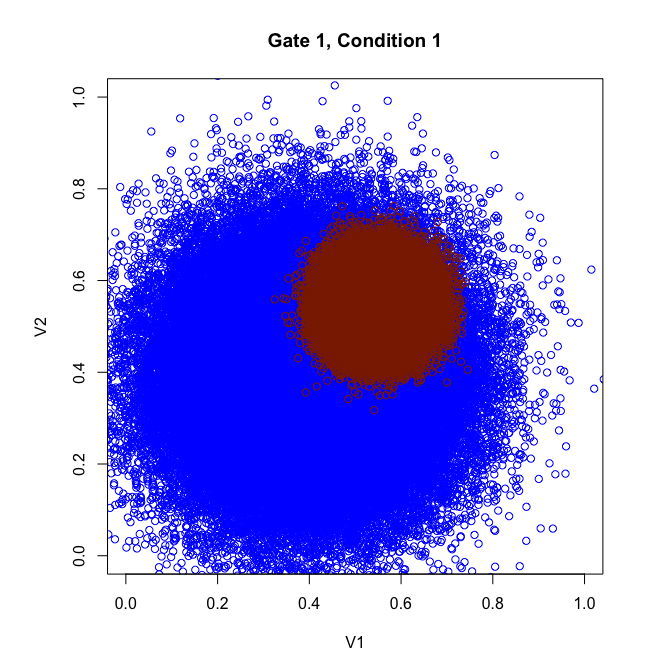At Condition 2, we generate 100,000 cases and 100,000 controls (ncell = 100000) randomly MVN with a case centroid at (0.45, 0.45) and a control centroid at (0.40, 0.40) within a unit square window (0, 1), and cases have a more focal cluster (scalar = 0.05) than controls (scalar = 0.10).

# Initial parameters
c2_cas_center <- c(0.45, 0.45)
c2_con_center <- c(0.40, 0.40)
# V1 and V2 at Condition 2
c2_cas <- rand_mvn(centre = c2_cas_center, ncell = ncell, scalar = 0.05)
c2_con <- rand_mvn(centre = c2_con_center, ncell = ncell, scalar = 0.10)
graphics::par(pty = "s")
graphics::plot(c2_con,
col = "cornflowerblue",
xlim = c(0, 1),
ylim = c(0, 1),
main = "Gate 1, Condition 2",
xlab = "V1",
ylab = "V2")
graphics::points(c2_cas, col = "orangered1")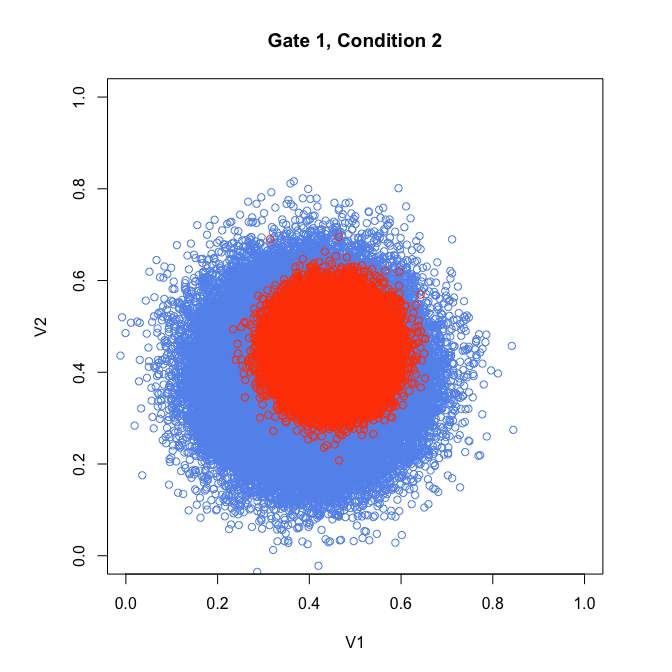# compile data
df_full <- tibble::tibble("id" = seq(1, ncell * 2 * 2, 1),
"group" = factor(c(rep("case", ncell * 2),
rep("control", ncell * 2))),
"condition" = factor(c(rep("2", ncell), rep("1", ncell),
rep("2", ncell), rep("1", ncell))),
"V1" = c(c2_cas[ , 1], c1_cas[ , 1], c2_con[ , 1], c1_con[ , 1]),
"V2" = c(c2_cas[ , 2], c1_cas[ , 2], c2_con[ , 2], c1_con[ , 2]))

rm(c2_cas, c1_cas, c2_con, c1_con) # conserve memory

#### Gate 2: Marker 3 and Marker 4

At Condition 1, we generate 100,000 cases and 100,000 controls (ncell = 100000) randomly MVN with a case centroid at (0.55, 0.55) and a control centroid at (0.50, 0.50) within a unit square window (0, 05), but both have the same amount of spread (scalar = 0.10).

# Initial parameters
c1_cas_center <- c(0.55, 0.55)
c1_con_center <- c(0.50, 0.50)
# V3 and V4 at Condition 1
c1_cas <- rand_mvn(centre = c1_cas_center, ncell = ncell, scalar = 0.05)
c1_con <- rand_mvn(centre = c1_con_center, ncell = ncell, scalar = 0.10)
graphics::par(pty = "s")
graphics::plot(c1_con,
col = "blue",
xlim = c(0, 1),
ylim = c(0, 1),
main = "Gate 2, Condition 1",
xlab = "V3",
ylab = "V4")
graphics::points(c1_cas, col = "orangered4")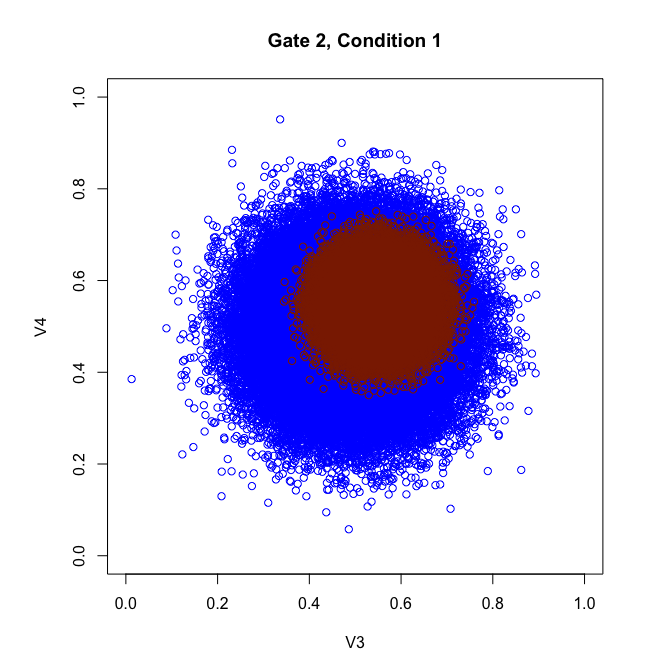At Condition 2, we generate 100,000 cases and 100,000 controls (ncell = 100000) randomly with a case centroid at (0.65, 0.65) and control a centroid at (0.50, 0.50) within a unit square window (0, 1), and cases have a more focal cluster (scalar = 0.05) than controls (scalar = 0.10).

# Initial parameters
c2_cas_center <- c(0.65, 0.65)
c2_con_center <- c(0.50, 0.50)
# V3 and V4 at Condition 2
c2_cas <- rand_mvn(centre = c2_cas_center, ncell = ncell, scalar = 0.05)
c2_con <- rand_mvn(centre = c2_con_center, ncell = ncell, scalar = 0.10)
graphics::par(pty = "s")
graphics::plot(c2_con,
col = "cornflowerblue",
xlim = c(0, 1),
ylim = c(0, 1),
main = "Gate 2, Condition 2",
xlab = "V3",
ylab = "V4")
graphics::points(c2_cas, col = "orangered1")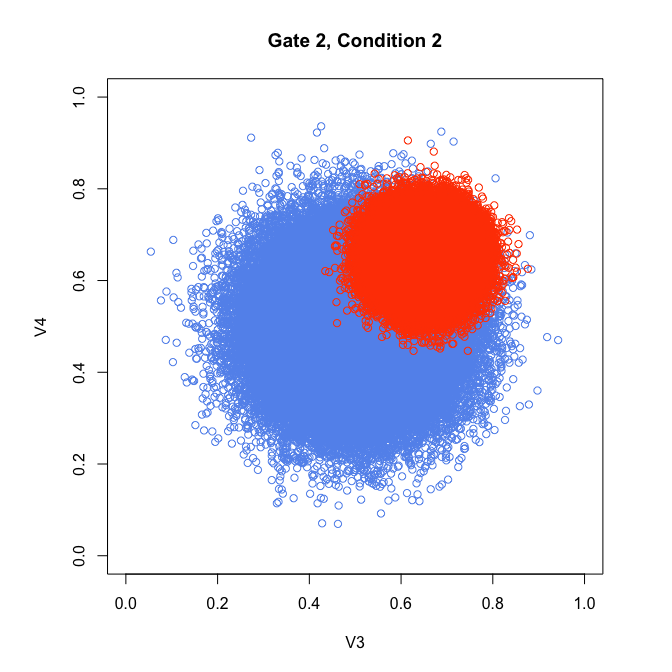Compile the toy data into a data frame

  df_full$V3 <- c(c2_cas[ , 1], c1_cas[ , 1], c2_con[ , 1], c1_con[ , 1]) df_full$V4 <-  c(c2_cas[ , 2], c1_cas[ , 2], c2_con[ , 2], c1_con[ , 2])

rm(c2_cas, c1_cas, c2_con, c1_con) # conserve memory

Generate random values for two example cytokines and append to the data frame.

# Two Cytokines
Z1 <- stats::rchisq(ncell * 4, df = 5) # Random Chi-square distribution
Z2 <- stats::rnorm(ncell * 4, 0, 1) # Random Gaussian distribution
# Append to data.frame
df_full$Z1 <- Z1 df_full$Z2 <- Z2
rm(Z1, Z2) # conserve memory
# Visualize histograms by the two group conditions
graphics::par(mfrow = c(2, 2), pty = "s")
graphics::plot(stats::density(df_full$Z1[df_full$group == "case"
& df_full$condition == "1"]), main = "Cytokine 1 of Cases at Condition 1") graphics::plot(stats::density(df_full$Z1[df_full$group == "case" & df_full$condition == "2"]),
main = "Cytokine 1 of Cases at Condition 2")
graphics::plot(stats::density(df_full$Z1[df_full$group == "control"
& df_full$condition == "1"]), main = "Cytokine 1 of Controls at Condition 1") graphics::plot(stats::density(df_full$Z1[df_full$group == "control" & df_full$condition == "2"]),
main = "Cytokine 1 of Controls at Condition 2")
graphics::plot(stats::density(df_full$Z2[df_full$group == "case"
& df_full$condition == "1"]), main = "Cytokine 2 of Cases at Condition 1") graphics::plot(stats::density(df_full$Z2[df_full$group == "case" & df_full$condition == "2"]),
main = "Cytokine 2 of Cases at Condition 2")
graphics::plot(stats::density(df_full$Z2[df_full$group == "control"
& df_full$condition == "1"]), main = "Cytokine 2 of Controls at Condition 1") graphics::plot(stats::density(df_full$Z2[df_full$group == "control" & df_full$condition == "2"]),
main = "Cytokine 2 of Controls at Condition 2")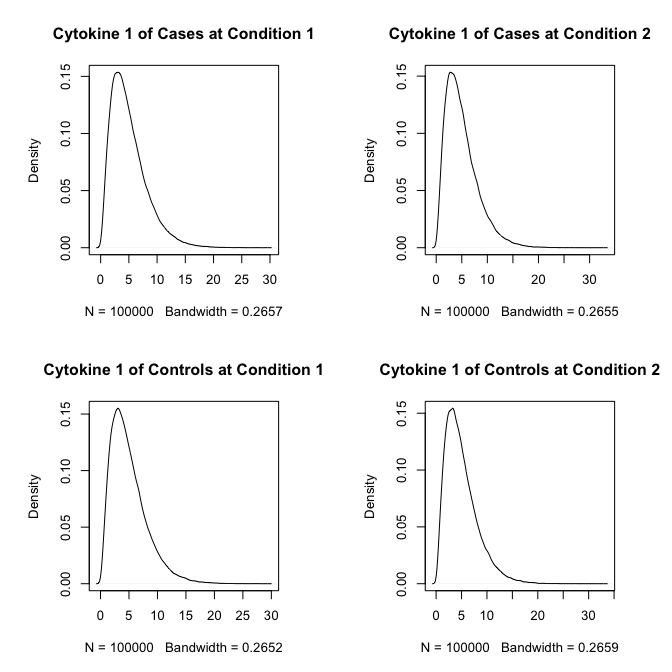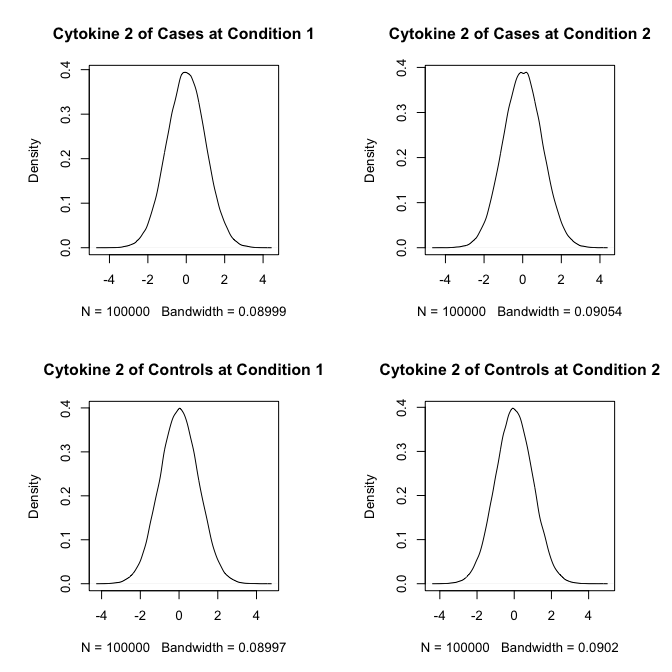The toy data frame has nine columns (id, groups, markers, and cytokines).

  utils::head(df_full)
## # A tibble: 6 x 9
##      id group condition    V1    V2    V3    V4    Z1      Z2
##   <dbl> <fct> <fct>     <dbl> <dbl> <dbl> <dbl> <dbl>   <dbl>
## 1     1 case  2         0.491 0.402 0.677 0.586  4.35 -0.488
## 2     2 case  2         0.407 0.493 0.714 0.698  8.61  0.279
## 3     3 case  2         0.508 0.409 0.547 0.644  6.79 -0.786
## 4     4 case  2         0.423 0.480 0.657 0.656  1.04 -0.552
## 5     5 case  2         0.367 0.420 0.635 0.637  4.10  0.239
## 6     6 case  2         0.499 0.405 0.547 0.656  6.99  0.0472

### For two conditions

# Initial parameters
alpha <- 0.05
vars <- c("V1", "V2", "V3", "V4")
p_correct <- "uncorrelated"
set.seed(1234) # for reproducibility
df_full <- as.data.frame(df_full)

# Gates 1 and 2
start_time <- Sys.time() # record start time
out_gate <- gateR::gating(dat = df_full,
vars = vars,
n_condition = 2,
doplot = TRUE,
alpha = alpha,
p_correct = p_correct,
c1n = "case", # level "case" as the numerator of first condition
c2n = "2") # level "2" as the numerator of second condition
end_time <- Sys.time() # record end time
total_time <- end_time - start_time # calculate duration of gating() example